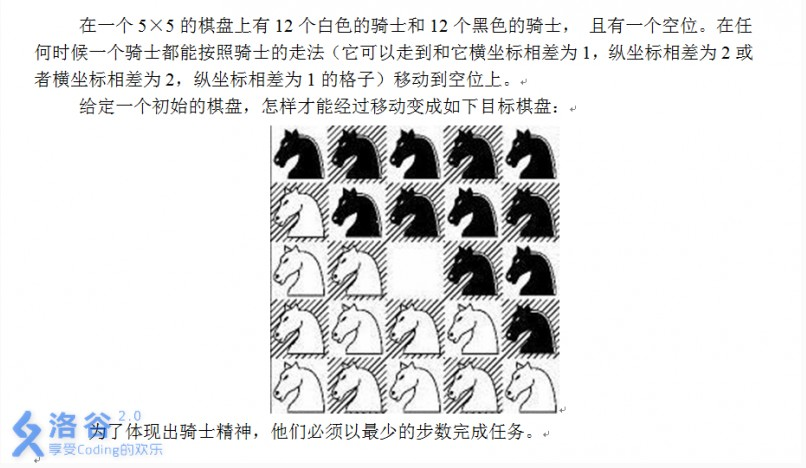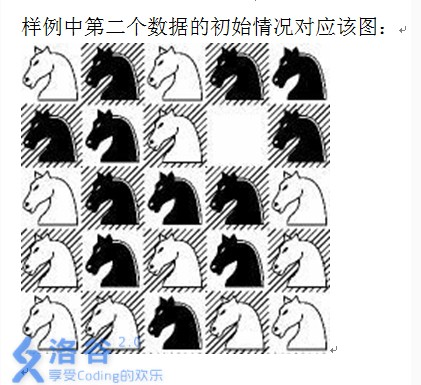# 洛谷 P2324 [SCOI2005]骑士精神 题解

2019/03/01 23:22

## 题目

### 题目描述### 输入输出样例

2
10110
01*11
10111
01001
00000
01011
110*1
01110
01010
00100


7
-1


### 说明## 考虑剪枝

1： 在搜索时记录与目标状态不同的不同的方格个数，因为除了最后一步可以一步将两个不正确的格子变为正确，其他时候每一步都只能将一个格子更正

if (now + ss - 1 > maxn) return false;  //剪枝 1 ：如果当前预计的最少步数已经超过限定的值，停止
//由于最后一步可以一下更正两格，所以最少步数为 now + ss - 1

2：不走回头路，如果走回去就continue

int dx = {-2, -2, -1, 1, -1, 1, 2, 2}, dy = {-1, 1, 2, 2, -2, -2, -1, 1};
//dx，dy按照一定的顺序来，便于判断是否走回去
for (int i = 0; i < 8; i++) //8个方向搜索
{
if (i + last == 7) continue;  //如果走第i个方向是往回走就不用搜索了（这就是为什么dx和dy要有一定顺序）

}

## 搜索方法

### 考虑空格

if (ch[xx][yy] == m[xx][yy] and ch[xx][yy] != m[x][y]) tmp++; //上述情况1
else if (ch[xx][yy] != m[xx][yy] and ch[xx][yy] == m[x][y]) tmp--; //情况2
if (m[x][y] == '*') tmp++; //情况3
else if (m[xx][yy] == '*') tmp--; //情况4

## 完整代码

#include <bits/stdc++.h>
using namespace std;
int t, sx, sy, s, maxn, ans;
int dx = {-2, -2, -1, 1, -1, 1, 2, 2}, dy = {-1, 1, 2, 2, -2, -2, -1, 1};
//dx，dy按照一定的顺序来，便于判断是否走回去
char ch;
char m={ {'1', '1', '1', '1', '1'},
{'0', '1', '1', '1', '1'},
{'0', '0', '*', '1', '1'},
{'0', '0', '0', '0', '1'},
{'0', '0', '0', '0', '0'}};
//打表便于对比状态
bool ok (int x, int y, int now, int ss, int last)
//x，y表示坐标，now表示当前步数，ss表示预计最少步数，last表示上一次走的方向
{
if (now + ss - 1 > maxn) return false;  //剪枝  ：如果当前预计的最少步数已经超过限定的值，停止
//由于最后一步可以一下更正两格，所以最少步数为 now + ss - 1
if (!ss) {ans = now;  return true;}  //如果当前状态就是目标状态，记录答案，返回true
for (int i = 0; i < 8; i++) //8个方向搜索
{
int tmp = ss;
if (i + last == 7) continue;  //如果走第i个方向是往回走就不用搜索了（这就是为什么dx和dy要有一定顺序）
int xx = x + dx[i], yy = y + dy[i];
if (xx < 0 or xx > 4 or yy < 0 or yy > 4) continue;
if (ch[xx][yy] == m[xx][yy] and ch[xx][yy] != m[x][y]) tmp++; //上述情况1
else if (ch[xx][yy] != m[xx][yy] and ch[xx][yy] == m[x][y]) tmp--; //情况2
if (m[x][y] == '*') tmp++; //情况3
else if (m[xx][yy] == '*') tmp--; //情况4
swap (ch[x][y], ch[xx][yy]);
bool k = ok (xx, yy, now + 1, tmp, i);
if (k) return true;
swap (ch[x][y], ch[xx][yy]); //回溯
}
return false;
}
int main()
{
scanf ("%d", &t);
while (t--)
{
s = 0, ans = -1;
for (int i = 0; i < 5; i++)
for (int j = 0; j < 5; j++)  {
cin >> ch[i][j];
if (ch[i][j] == '*') sx = i, sy = j;
if (ch[i][j] != m[i][j]) s++; //如果和目标不同，统计加 1
}
for (int i = s; i <= 15; i++){
maxn = i;
if (ok (sx, sy, 0, s, 10))  break;
}
printf ("%d\n", ans);
}
return 0;
}

0
0 收藏

### 作者的其它热门文章0 评论
0 收藏
0